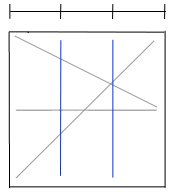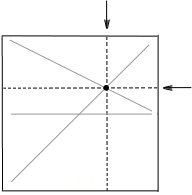# Divide Paper into Thirds: Method 3There are many ways to divide a sheet of paper into thirds, some methods are easier than others. The method shown here is:
– fairly easy,
– but will leave 3 crease marks with one crease along the diagonal (which may or may not be useful later).#### Divide Paper into Thirds: Method 3

• Fold and unfold a square sheet of paper top to bottom.

• Fold and unfold along the diagonal.

• Fold and unfold the top right corner so the crease extends from from top-left corner to where horizontal crease line meets right edge of paper.

• The location where the two creases intersect is the division of 1/3 and 2/3. Fold along this point of intersection to get 1/3 division.

• Fold the section that is 2/3 wide in half to get 1/3 + 1/3 + 1/3 divisionNote: the point where the two lines intersect shows the 1/3 division along the vertical and the horizontal. Thus, a vertical crease or a horizontal crease which passes through the dot gives 1/3 division.